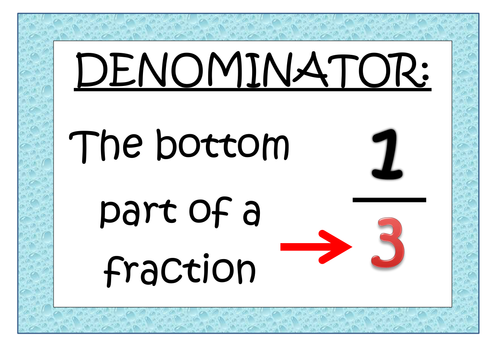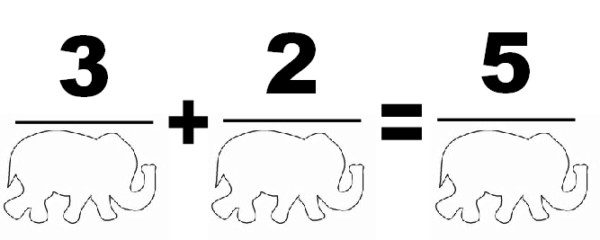* indicates a required field• phone number format invalid• email format invalidProblems detected, please review the form.
Privacy - Terms

### Why do kids fear and struggle with fractions?

May 7, 2018Many kids fear fractions because they don’t understand how they work – they mix up the parts and don’t understand what they mean and what we do to them.

Parents typically understand enough to get by in everyday life (1/2 off sale – 3/4” wrench – 1/4 tank of gas), but have a difficult time explaining fractions in a way that helps kids understand. In fact, most of us struggled with fractions as kids! So it makes sense that we can’t explain it to our kids.

Another common error we make as parents is that when we understand something, we still may not be able to explain it well. We can make some assumptions while we are explaining things and get frustrated when kids don’t comprehend it. It’s like playing Pictionary, and your team gives you the “blank stare.”

We also often find that kids fear fractions because of older siblings. If kids see their older brother or sister struggle with fractions, they automatically assume it’s going to happen to them. They have perceived fractions as being too hard for them before even having the chance to try.

So how can you fix this? How can you explain this so they get it?

Let’s start at the beginning. What is a Fraction?

It’s just breaking something whole into smaller equal parts.

When we start working with young students, we talk about the concept of half.  What is half?  What exactly does it mean?  We take for granted the meaning of these words and assume that kids understand them.  But how would you explain the meaning of the word "half" to a 5 year old without using the word “half” itself?

We know that most kids have had to share at some point in their lives.  They know how much half a cookie is.  They know that when you share something with one other person, the 2 pieces should be the same size (equal).  Knowing that they understand this concept, we can build on it. If half is 2 parts that are the same size, then thirds is 3 parts the same size, and so on.   Even though it sounds simple, this forms the basic construct for fractions.

Next you need to understand the parts of the fraction.

Let’s start with the Bottom Number. What is it, and why is it there?The Bottom Number, or the Denominator, is the “name” of the fraction. The root of the word, -nom-, actually means “name.” Words are important in math, too!

So the Denominator tells us 2 things: how many parts our whole is divided into, and what to call these parts.  When we see a 4 in the Denominator of a fraction, it means we have broken something into 4 equal parts, and we call those parts "Fourths."

The top number, or the Numerator, is the number of those parts we have.  When we see the fraction 3/4, it tells us that we have 3 out of the 4 equal parts.

At this point, you need to remember that there is a fine line between the Numerator and the Denominator.  Only 3 out of 2 people will get that fraction joke! (Those are the only two Fractions jokes I know.)

So why do you need Common Denominators when adding or subtracting fractions?

The explanation is very simple when you remember that the Denominator simply means, “The name of the fraction”. It tells you what you’re dealing with. If you buy 3 apples, then you buy 2 more apples, together you bought 5 apples.

3/apples +2/apples = 5/apples

However, if you buy 3 apples and 2 bananas, you can’t add them because they aren’t the same thing. You don’t have 5 banapples! You can’t add or subtract things with different names. You have to find a common “name” for these items.

So if you change the “name” to fruit – it works!

3/fruit + 2/fruit = 5/fruit!

Fractions work the same way!

apples plus 2 apples equals 5 apples.

– Or –elephants plus 2 elephants equals 5 elephants.

– Or –

sevenths (3/7) plus 2 sevenths (2/7) equals 5 sevenths (5/7).

You have to think of the Denominator not as a number, but as a “name.”

But what if we are adding two things that don’t have the same name?

3 apples + 2 bananas

3 pennies + 2 nickels

You don’t get 5 banapples or 5 pickles do you?

No, you change the names so they are the same.  You have 5 fruit and 13 cents.

So if we have 1 third plus 1 half (1/3 + 1/2), we can’t add them, because the names are different. Just like if we had 1 dime and 1 nickel, those aren't the same type of coin. But if we turn them both into cents first, we can add them together and get 15 cents! With fractions, just like with coins, when you change the name, the number changes too. With 1/3 and 1/2, the common name is sixths, and 1/3 = 2/6, while 1/2 = 3/6. So we now have 2 sixths plus 3 sixths equals 5 sixths (2/6 + 3/6 = 5/6).

This is how we teach at Mathnasium.  We break down concepts into the simplest terms and use analogies like these, so your kids can understand how and why the concepts work.  We don’t want to just teach rules and algorithms that they are going to forget.

What about Equivalent Fractions? What about Multiplying and Dividing Fractions? Reducing Fractions?

We teach these topics and concepts in a similar way so that kids understand what to do, and why they are doing it. This is the best teaching method for long term retention. It's easy to forget something you've memorized, but it's hard to forget something that you truly understand!

Mathnasium’s goal for teaching fractions is to help the student master them. This conquers the fear and builds confidence! We help them to think and process mathematical concepts the way natural mathematicians do, so that they can work confidently at a high level.To learn more how Mathnasium can help fix your child's fractured learning of fractions, contact us (708) 966 9879 or via email at orlandpark@mathnasium.com.